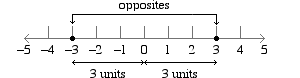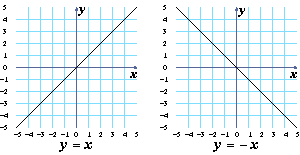# Absolute Value

In this lesson you will learn about absolute values of numbers, and study graphs of formulas involving absolute value. You will also learn how to solve equations involving absolute value.

As you know, every real number except zero has an opposite, and two numbers that are opposites of each other are the same distance from zero. For example, \$-3\$ and 3 are opposites, and each is 3 units away from zero.The absolute value of a number is the distance between that number and zero. For example, the absolute value of 3 is 3 and the absolute value of \$-3\$ is 3. The absolute value of 3 is written \$|3|\$, so \${|3|}=3\$ and \${|-3|}=3\$.

Use the number line above to find the absolute value of each number in this table.
numberabsolute value
 What numbers are 7 units away from zero? and
 What numbers have an absolute value of 7? and
 If \${|x|}=7\$, what are the possible values of \$x\$? and
 If \${|x|}=10\$, what are the possible values of \$x\$? and

## The graph of \$y={|x|}\$

Complete this table for the equation \$y={|x|}\$.
\$x\$\$y\$

Click to see the graph of \$y={|x|}\$.

 Is \$y\$ ever positive?
 Is \$y\$ ever zero?
 Is \$y\$ ever negative?

Look at the graphs of \$y=x\$ and \$y=-x\$ shown below. Compare the graph of \$y={|x|}\$ shown on the grid to the left to these graphs.If you only look at \$x\$-values that are greater than zero, does \$y={|x|}\$ look like \$y=x\$ or \$y=-x\$?
 If you only look at \$x\$-values that are less than zero, does \$y={|x|}\$ look like \$y=x\$ or \$y=-x\$?

Look at the graph of \$y={|x|}\$. On this graph, there are two points that have a \$y\$-value of 2: the points \$(2,2)\$ and \$(-2,2)\$. This means that if \${|x|}=2\$, then \$x\$ can be either 2 or \$-2\$.

 If \${|x|}=4\$, what can \$x\$ be? \$x\$ = or
 If \${|x|}=0\$, what can \$x\$ be? \$x\$ =
 Can \${|x|}=-2\$?

Look at the graph of \$y={|x|}\$, and answer the following questions about its shape and properties.

 Which letter of the alphabet is the graph shaped like?
 Which two quadrants of the coordinate grid is the graph in? (Remember that quadrants start in the upper right quarter of the grid and are numbered counterclockwise.)
 For a particular positive \$y\$-value, how many points on the graph have that \$y\$-value?
 For a particular negative \$y\$-value, how many points on the graph have that \$y\$-value?

## Solving equations involving absolute value

You have seen that if \${|x|}=3\$, \$x\$ can be either 3 or \$-3\$ because both 3 and \$-3\$ are 3 units away from zero. In this section you will learn how to solve equations such as \${|x-2|}=3\$. First, let’s see what the graph of \$y={|x-2|}\$ looks like.

Complete this table for \$y={|x-2|}\$.
\$x\$\$y\$
 Are any of the \$y\$-values in the table negative?
 Give two \$x\$-values in the table which have the same \$y\$-value. and

Click to look at the graph of \$y={|x-2|}\$.

 What letter is the graph of \$y={|x-2|}\$ shaped like?
 Does the graph of \$y={|x-2|}\$ ever go below the \$x\$-axis?
 At what point \$(x, y)\$ does the graph of \$y={|x-2|}\$ touch the \$x\$-axis?

On the graph of \$y={|x-2|}\$ there are two points that have a \$y\$-value of 3: the points \$(-1,3)\$ and \$(5,3)\$. This means that if \${|x-2|}=3\$, then \$x\$ can be either \$-1\$ or 5. Use the graph to answer the following questions.

 If \${|x-2|}=1\$, what can \$x\$ be? \$x\$ = or
 If \${|x-2|}=0\$, what can \$x\$ be? \$x\$ =
 Can \${|x-2|}=-1\$?

The graph of \$y={|x-2|}\$ is shown in green and the graph of \$w={|x|}\$ is shown in red.

 Are the two graphs the same shape, or different shapes?
 Is the graph of \$y={|x-2|}\$ above the graph of \$w={|x|}\$, below the graph of \$w={|x|}\$, to the left of that graph, or to the right of that graph?
 How many units in that direction is the graph of \$y={|x-2|}\$ from the graph of \$w={|x|}\$?

You can also solve equations involving absolute value algebraically. You know that if \${|x|}=3\$, then \$x=3\$ or \$x=-3\$. Similarly, if \${|x-2|}=3\$, then \$x-2\$ must equal 3 or \$-3\$.

 \$\${|x-2|}=3\$\$ \$\$\cl"tight"{\table x, -, 2, , , =, 3; x, -, 2, +, 2, =, 3, +, 2; x, , , , , =, \colspan 3 {5}}\$\$ OR \$\$\cl"tight"{\table x, -, 2, , , =, -3; x, -, 2, +, 2, =, -3, +, 2; x, , , , , =, \colspan 3 {-1}}\$\$

Always check your solutions by substituting them back in the original equation:

Test \$x=5\$: \${|5-2|}={|3|}=3\$. True.

Test \$x=-1\$: \${|-1-2|}={|-3|}=3\$. True.

Solve each equation in the table below algebraically, as shown above. Check your solutions by substituting them in the original equation.

## More graphs involving absolute value

The equations \$y=-\,{|x|}\$ (in blue) and \$w={|x|}\$ (in red) are graphed on the grid to the left.

 Are the two graphs the same shape as each other?
 Do they point in the same direction as each other?
 If you wanted to get the red graph to lie exactly on top of the blue graph, which axis would you have to flip it over? The -axis
 The purple line on the bottom grid is the graph of \$y=a\,{|x-h|}+k\$. Use the sliders to find an equation for the graph shown on the top grid. The equation for your purple graph is shown below the grids.
 When does \$y\$ have the value \$-2\$? \$x\$ = or

## Multiplying or adding absolute values

Compute \${|a|}\,{|b|}\$ and \$|ab|\$ for the values of \$a\$ and \$b\$ in the table below.

\$a\$\$b\$\${|a|}\,{|b|}\$\$|ab|\$

The equations \$y={|a|}\,{|x|}\$ and \$y={|ax|}\$ are graphed on the grids to the left, with a slider for \$a\$.

 Do the graphs of those equations look the same or different?
 Does the answer to your last question change as you slide the slider for \$a\$?

As you can see:

For any two numbers \$a\$ and \$b\$, \${|a|}\,{|b|}={|ab|}\$.

In the last question, you saw that \${|a|}\,{|b|}={|ab|}\$. That is, it doesn’t matter whether you multiply two numbers first or take their absolute value first; the end result is the same either way. In this question, we’ll look at sums of absolute values.

Find two numbers \$a\$ and \$b\$ so that \$|a|+{|b|}\$ is not equal to \$|a+b|\$. (Try mixing positive and negative numbers.)

The equations \$y={|x|}+{|a|}\$ and \$y={|x+a|}\$ are graphed on the grids to the left, with a slider for \$a\$.

 As you change \$a\$, are the two graphs the same or different?